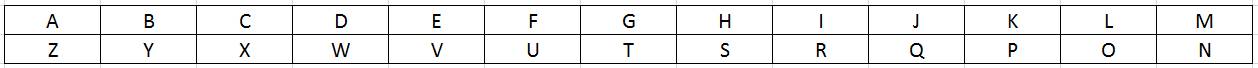0
174

# SSC CHSL Expected Questions

SSC CHSL Expected Questions download PDF based on previous year question paper of SSC exams. 20 Very important Expected questions for SSC CHSL Exam.

Question 1: Three positions of a cube are given below

a) 1

b) 2

c) 3

d) 4

Question 2: Three positions of a cube are given below

a) 1

b) 2

c) 3

d) 4

Question 3: Three positions of a cube are given below

a) 1

b) 2

c) 3

d) 4

Question 4: Three positions of a cube are given below

a) 1

b) 2

c) 3

d) 4

Question 5: Which of the following cube in the answer figure cannot be made based on unfolded cube in the question figure?

a)

b)

c)

d)

SSC CHSL Study Material (FREE Tests)

Question 6: What is the next term in the series ?
12,12,36,180,?

a) 1060

b) 1450

c) 1320

d) 1260

Question 7: What is the next term in the series ?
6,24,60,120,?

a) 150

b) 180

c) 210

d) 240

Question 8: What is the next term in the series ?
5040,720,145,45,?

a) 15

b) 5

c) 30

d) 45

Question 9: If HUT is coded as 3360 and TAP is coded as 320, then PUN is coded as?

a) 4304

b) 3904

c) 4704

d) 3604

Question 10: If 9 & 7 # 6 = 51 and 12 & 7 # 9 = 75, then find the value of 23 & 6 # 7

a) 72

b) 69

c) 65

d) 63

Instructions

Which of the following signs should be interchanged so that the given equation is true?

Question 11: $24-120+30\times4\div8$=16

a) $\times$,+

b) $\times$,$\div$

c) +,$\div$

d) -,+

Question 12: $87+12\div3-248\times50$=150

a) $\times$,+

b) $\times$,$\div$

c) -,$\div$

d) -,+

Question 13: Eye:Ophthalmology::Liver:?

a) Osteology

b) Dermatology

c) Hepatology

d) Nephrology

Question 14: Kidney:Nephrology::Bones:?

a) Ophthalmology

b) Hepatology

c) Osteology

d) Dermatology

Question 15: Waves:Oceans::?:Deserts

a) Dust

b) Storms

c) Ripples

d) Sand Dunes

Question 16: If $\sec \theta +\tan \theta=2$, then the value of $\sin \theta$ is:

a) $\frac{4}{5}$

b) $\frac{\sqrt{3}}{5}$

c) $\frac{2}{5}$

d) $\frac{3}{5}$

Question 17: If $\alpha + \theta$ =  $\frac{7\pi}{12}$ and $\tan \theta = \sqrt{3}$, then the value of $\tan \alpha$ is:

a) $\sqrt{3}$

b) 1

c) 0

d) $\frac{1}{\sqrt{3}}$

Question 18: A person sells 40 chairs for Rs 10000 and gets a loss of 4% and if he had sold 30 chairs for Rs 10000 what would be his profit or loss percent ?

a) 24%

b) 28%

c) 26%

d) 22%

Question 19: Kavya buys 200 erasers at Rs 25 each and she sells 100 erasers at 10% profit. If the total profit to be obtained is 20% then the other 100 erasers should be sold at which price per eraser ?

a) 32

b) 32.5

c) 32.75

d) 32.25

Instructions

Out of the given alternatives, select the option which can be substituted in place of the given words without altering the meaning.

Question 20: One who does not follow the usual way of life

a) Bohemian

b) Codicil

c) Dereliction

d) Efface

When we fold the given figure, the circle with a star sign will become the base of the cube and the side with window sign will become top, and thus they will face each other. Similarly, the sides which will face each other will be :

Since, the opposite sides cannot be adjacent in the cube, thus the cube in the last option is not possible.

=> Ans – (D)

In this series
12*1=12
12*3=36
36*5=180
180*7=1260

In this series
1*2*3=6
2*3*4=24
3*4*5=60
4*5*6=120
5*6*7=210

In this series next number is obtained by dividing the previous number by 7,5,3,1
5040/7=720
720/5=145
145/3=45
45/1=45

Let us write the alphabets from A to Z in two rows as shown below.

1            2             3             4             5             6             7             8               9            10            11          12           1326          25           24            23             22            21           20           19            18           17           16           15          14

Given,

HUT = 3360

H = 8; U = 21; T = 20

HUT = $8 \times 21 \times 20 = 3360$

TAP is coded as 320

T = 20; A=1; P = 16

TAP = $20 \times 1 \times 16 = 320$

Similarly,

PUN = $16 \times 21 \times 14 = 4704$

Given, 9 & 7 # 6 = 51 and 12 & 7 # 9 = 75

Here the symbol $represents +, and the symbol # represents$\times$9 + 7$\times$6 = 51 12 + 7$\times$9 = 75$\therefore$23 + 6$\times$7 = 65 11) Answer (C) After interchanging the signs + and$\div$we get$24-120\div30\times4+8$=24-16+8 =16 12) Answer (A) After interchanging the signs + and \times we get$87\times12\div3-248+50$=348-248+50 =150 13) Answer (C) Study of Eye is known as Ophthalmology.Study of Liver is Hepatology. 14) Answer (C) Study of kidney is known as Nephrology. Study of Bones is Osteology. 15) Answer (D) In Oceans we get Waves and similarly in Deserts we get Sand Dunes. 16) Answer (D) Given :$\sec \theta +\tan \theta=2$———–(i) Also,$sec^2\theta-tan^2\theta=1$=>$(sec\theta-tan\theta)(sec\theta+tan\theta)=1$=>$\sec \theta -\tan \theta=\frac{1}{2}$———-(ii) Adding equations (i) and (ii), =>$2sec\theta=2+\frac{1}{2}=\frac{5}{2}$=>$sec\theta=\frac{5}{4}$=>$cos\theta=\frac{4}{5}\thereforesin\theta=\sqrt{1-cos^2\theta}$=$\sqrt{1-(\frac{4}{5})^2}=\sqrt{1-\frac{16}{25}}$=$\sqrt{\frac{9}{25}}=\frac{3}{5}$=> Ans – (D) 17) Answer (B) Given :$\tan \theta = \sqrt{3}$=>$\tan \theta = tan(\frac{\pi}{3})$=>$\theta=\frac{\pi}{3}$Also,$\alpha + \theta$=$\frac{7\pi}{12}$=>$\alpha=\frac{7\pi}{12}-\frac{\pi}{3}$=>$\alpha=\frac{7\pi-4\pi}{12}=\frac{\pi}{4}\thereforetan\alpha=tan(\frac{\pi}{4})=1\$

=> Ans – (B)

SP of 40 chairs =10000
SP of 1 chair=250
Given a loss of 4% and so CP of 1 chair=250/0.96
CP of 30 chairs=(250/0.96)*30
CP of 30 chairs=7812.5
Profit percent=((1000-7812.5)/7812.5)*100
=28%